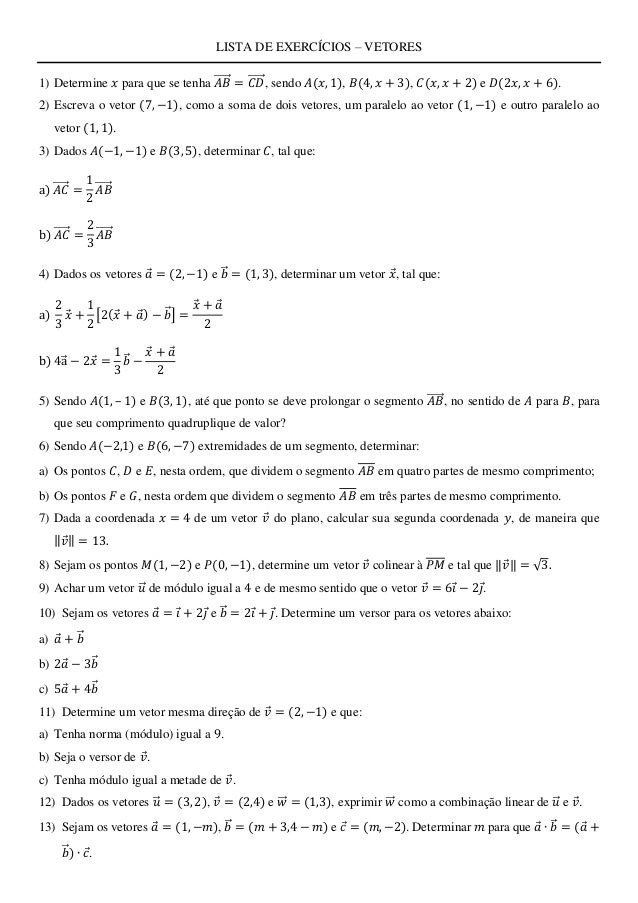Successfully reported this slideshow.
We use your LinkedIn profile and activity data to personalize ads and to show you more relevant ads. You can change your ad preferences anytime.Upcoming SlideShare
Loading in …5
×

# Lista de exercícios - vetores(Alguns gabaritos estão errados)

18 views

Published on

Lista de exercícios ofertada pelo professor Deivson Sales à turma TM de Eng. de Computação - POLI (UPE).

Bons estudos. Deus te abençoe!

Published in: Education
• Full Name
Comment goes here.

Are you sure you want to Yes No
Your message goes here• Be the first to comment

• Be the first to like this

### Lista de exercícios - vetores(Alguns gabaritos estão errados)

1. 1. LISTA DE EXERCÍCIOS – VETORES 1) Determine 𝑥 para que se tenha 𝐴𝐵⃗⃗⃗⃗⃗ = 𝐶𝐷⃗⃗⃗⃗⃗ , sendo 𝐴(𝑥, 1), 𝐵(4, 𝑥 + 3), 𝐶(𝑥, 𝑥 + 2) e 𝐷(2𝑥, 𝑥 + 6). 2) Escreva o vetor (7, −1), como a soma de dois vetores, um paralelo ao vetor (1, −1) e outro paralelo ao vetor (1, 1). 3) Dados 𝐴(−1, −1) e 𝐵(3, 5), determinar 𝐶, tal que: a) 𝐴𝐶⃗⃗⃗⃗⃗ = 1 2 𝐴𝐵⃗⃗⃗⃗⃗ b) 𝐴𝐶⃗⃗⃗⃗⃗ = 2 3 𝐴𝐵⃗⃗⃗⃗⃗ 4) Dados os vetores 𝑎 = (2, −1) e 𝑏⃗ = (1, 3), determinar um vetor 𝑥, tal que: a) 2 3 𝑥 + 1 2 [2(𝑥 + 𝑎) − 𝑏⃗ ] = 𝑥 + 𝑎 2 b) 4a⃗ − 2𝑥 = 1 3 𝑏⃗ − 𝑥 + 𝑎 2 5) Sendo 𝐴(1, – 1) e 𝐵(3, 1), até que ponto se deve prolongar o segmento 𝐴𝐵⃗⃗⃗⃗⃗ , no sentido de 𝐴 para 𝐵, para que seu comprimento quadruplique de valor? 6) Sendo 𝐴(−2,1) e 𝐵(6, −7) extremidades de um segmento, determinar: a) Os pontos 𝐶, 𝐷 e 𝐸, nesta ordem, que dividem o segmento 𝐴𝐵 em quatro partes de mesmo comprimento; b) Os pontos 𝐹 e 𝐺, nesta ordem que dividem o segmento 𝐴𝐵 em três partes de mesmo comprimento. 7) Dada a coordenada 𝑥 = 4 de um vetor 𝑣 do plano, calcular sua segunda coordenada 𝑦, de maneira que ‖ 𝑣‖ = 13. 8) Sejam os pontos 𝑀(1, −2) e 𝑃(0, −1), determine um vetor 𝑣 colinear à 𝑃𝑀 e tal que ‖ 𝑣‖ = √3. 9) Achar um vetor 𝑢⃗ de módulo igual a 4 e de mesmo sentido que o vetor 𝑣 = 6𝑖 − 2𝑗. 10) Sejam os vetores 𝑎 = 𝑖 + 2𝑗 e 𝑏⃗ = 2𝑖 + 𝑗. Determine um versor para os vetores abaixo: a) 𝑎 + 𝑏⃗ b) 2𝑎 − 3𝑏⃗ c) 5𝑎 + 4𝑏⃗ 11) Determine um vetor mesma direção de 𝑣 = (2, −1) e que: a) Tenha norma (módulo) igual a 9. b) Seja o versor de 𝑣. c) Tenha módulo igual a metade de 𝑣. 12) Dados os vetores 𝑢⃗ = (3, 2), 𝑣 = (2,4) e 𝑤⃗⃗ = (1,3), exprimir 𝑤⃗⃗ como a combinação linear de 𝑢⃗ e 𝑣. 13) Sejam os vetores 𝑎 = (1, −𝑚), 𝑏⃗ = (𝑚 + 3,4 − 𝑚) e 𝑐 = (𝑚, −2). Determinar 𝑚 para que 𝑎 ∙ 𝑏⃗ = (𝑎 + 𝑏⃗ ) ∙ 𝑐.
2. 2. 14) Os vetores 𝑎 e 𝑏⃗ formam um ângulo de 150°. Sabe-se que ‖ 𝑎‖ = √3 e ‖𝑏⃗ ‖ = √2, calcule: a) ‖𝑎 + 𝑏⃗ ‖ b) ‖𝑎 − 𝑏⃗ ‖ c) ‖3𝑎 + 2𝑏⃗ ‖ d) ‖5𝑎 − 4𝑏⃗ ‖ 15) Determine um vetor unitário ortogonal aos vetores 𝑎 = (2, 6) e 𝑏⃗ = (0, −2). 16) O vetor 𝑣 = (−1, −1) forma um ângulo de 60° com um vetor 𝐴𝐵⃗⃗⃗⃗⃗ , onde 𝐴(0, 3) e 𝐵 = (𝑚, −1). Calcular o valor de 𝑚. 17) Dados os vetores 𝑢⃗ = (2, −3) e 𝑣 = (3, −4), determine: a) Projeção de 𝑢⃗ em 𝑣. b) Projeção de 𝑣 em 𝑢⃗ . SOLUÇÃO 1) 𝑥 = 2 2) (7, −1) = 4(1, −1) + 3(1, 1) 3) a) 𝐶 = (−3, −4) b) 𝐶 = (−11/3, −5) 4) a) 𝑥 = (−3/7, 12/7) b) 𝑥 = (52/9, −11/3) 5) (9, 7) 6) a) 𝐶 = (0, −1), 𝐷 = (2, −3), 𝐸 = (4, −5) b) 𝐹 = (2/3, −5/3), 𝐷 = (10/3, −13/3) 7) 𝑦 = ±3√17 8) 𝑣 = (√3/2, −√3/2) 9) 𝑢⃗ = (6√10/5, −6√10/5) 10) a) (1/√2, 1/√2) b) (−4/√17, 1/√17) c) (13/√365, 14/√365) 11) a) (18√5/5, −9√5/5) b) (2/√5, −1/√5) c) (1, −1/2) 12) (1, 3) = (−1/4)(3, 2) + (7/8)(2, 4) 13) 𝑚 = 1 14) a) √5 − √18/2 b) √5 + √18/2 c) √25 − 3√18 d) √112 + 10√18 15) Não existe 16) 𝑥 = 1/3 (16 − 2√2 − √78 − 16√2) 17) a) (36/13, −54/13) b) (54/25, −72/25)